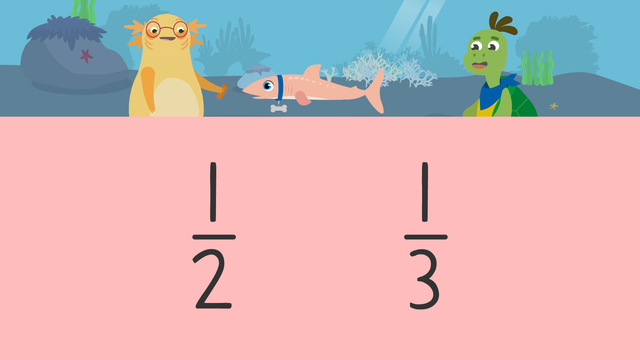# Fractions with the Same NumeratorRate this video

Ø 5.0 / 1 ratings

The authorTeam Digital
Fractions with the Same Numerator
CCSS.MATH.CONTENT.3.NF.A.3.D

## Information about the videoFractions with the Same Numerator

### In this Video about Comparing Fractions with the Same Numerator

Axel and Tank are training Tank’s new pet dogfish, Sparky. They each break the same-sized treat into pieces. They think they are feeding Sparky the same amount because they each feed him the same number of pieces. However, they divided their treats into different amounts. In this video, we learn to compare fractions with the same numerator to decide who actually gave Sparky more.

### Fractions with the Same Numerator

Fractions with the same numerator have the same number of shaded parts, but may have different denominators. As such, we will need to compare them closely. When the whole is the same, a smaller number in the denominator means the pieces will be larger since there is less to divide between.

#### How Can I Compare Two Fractions with the Same Numerator?

Let’s explore how to compare fractions with the same numerator. We can use fraction bars to compare the values of fractions with the same numerator. Let's use the fractions one-half and one-third as examples. Both fractions come from an equal-sized whole. They also both have the same numerator: one. However, their denominators are different.Now we can explain how to compare fractions with the same numerator but different denominators. Draw a fraction bar to represent the first fraction. Next, shade the bar to represent one-half.Then, draw a fraction bar of identical size to represent the second fraction. Now, shade the bar to represent one-third.Finally, compare the shaded parts of both models.One-half has a denominator that is smaller than one-third, but as we can see the whole is divided into just two parts so the pieces are actually larger. When comparing fractions with the same numerator and the same whole, the one with the smaller denominator is actually larger.### Summary of Steps: Comparing Fractions with the Same Numerator

To compare the size of two fractions with the same whole and the same numerator...

• First, draw and shade a fraction bar to represent the first fraction.

• Then, draw and shade a fraction bar of identical size to represent the second fraction.

• Finally, compare the shaded parts of both bars.

*If two fractions with the same whole have the same numerator, but different denominators, the fraction with the smaller denominator is actually larger.

### Comparing Fractions With Same Numerator: Third Grade Activities

Have you practiced yet? On this website, you can also find comparing fractions with the same numerator worksheets and exercises.